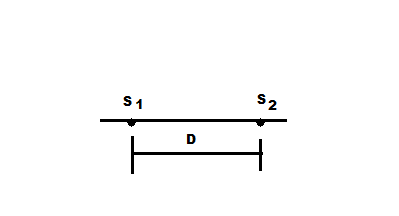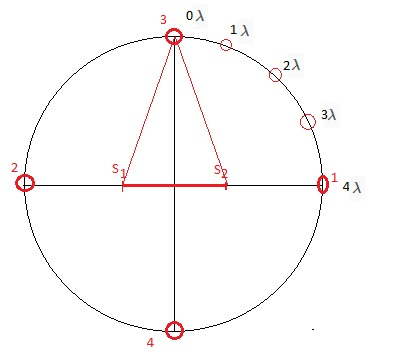# The figure shows two isotropic point sources of sound S_1 and S_2. The sources emit waves in...

## Question:

The figure shows two isotropic point sources of sound {eq}S_1 {/eq} and {eq}S_2 {/eq}. The sources emit waves in phase at wavelength 0.5 m: they are separated by D = 2.00 m. If we move a sound detector along a large circle centered at the midpoint between the sources, at how many points do waves arrive at the detector (a) exactly in phase and (b) exactly out of phase?## Constructive and Destructive Interference:

The point at which the wave meets in the phase, then the amplitude of the resultant wave increases due to superposition, and this process is known as constructive interference. And the destructive interference takes place when the wave meets are out of phase. Therefore, for the constructive interference, the path difference of the wave must be an integral multiple of the wavelength. The mathematical expression for the constructive interference is given by

{eq}\rm \delta L = n \lambda {/eq}

where

• {eq}\rm \delta L {/eq} is the path difference
• {eq}\rm n = 1, 2, 3, ..... {/eq} are the integers
• {eq}\rm \lambda {/eq} is the wavelength,

Similarly for the destructive interference

{eq}\rm \delta L = \left(n - \dfrac{1}{2}\right)\lambda {/eq}Given data:

• Distance between the source{eq}\rm (D) = 2.00 \ m {/eq}
• Wavelength of the source {eq}\rm (\lambda) = 0.5 \ m {/eq}

The difference in the two sources is of

{eq}\rm \dfrac{D}{\lambda} = \dfrac{2}{0.5} \\ D = 4 \lambda {/eq}

Therefore, at the horizontal axis, as shown in the figure, points would be of constructive interference because the path difference would be equal to the integral multiple of the wavelength.

At point 1, the path difference would be

{eq}\rm \delta L = S_{2}1 - S_{1}1 \\ \delta L = D \\ \delta L = 4 \lambda {/eq}

At point 2,

{eq}\rm \delta L = S_{2}2 - S_{1}2 \\ \delta L = D \\ \delta L = 4 \lambda {/eq}

therefore, the constructive interference takes place.

Similarly, the points on the vertical axis will have no path difference, therefore constructive interference takes place.

(a)

Therefore, there would be four points in one quadrant where the constructive interference takes place,

Hence the total 16 points, will be of constructive interference.

(b)

Similarly, there would be 16 points where destructive interference will take place due to symmetry.